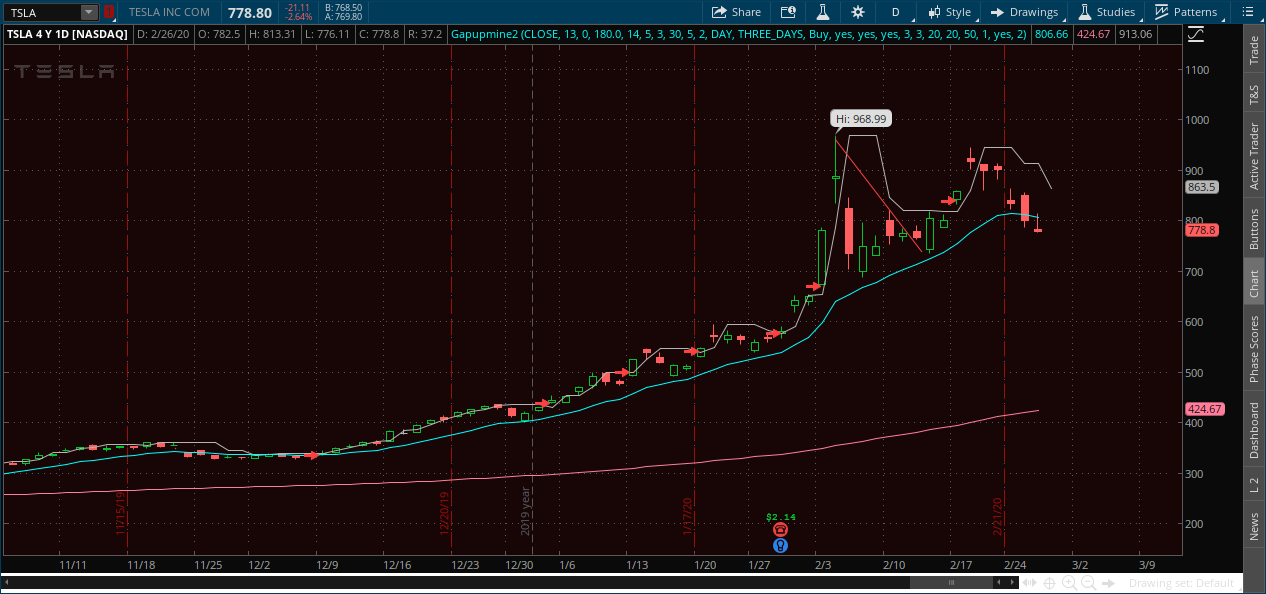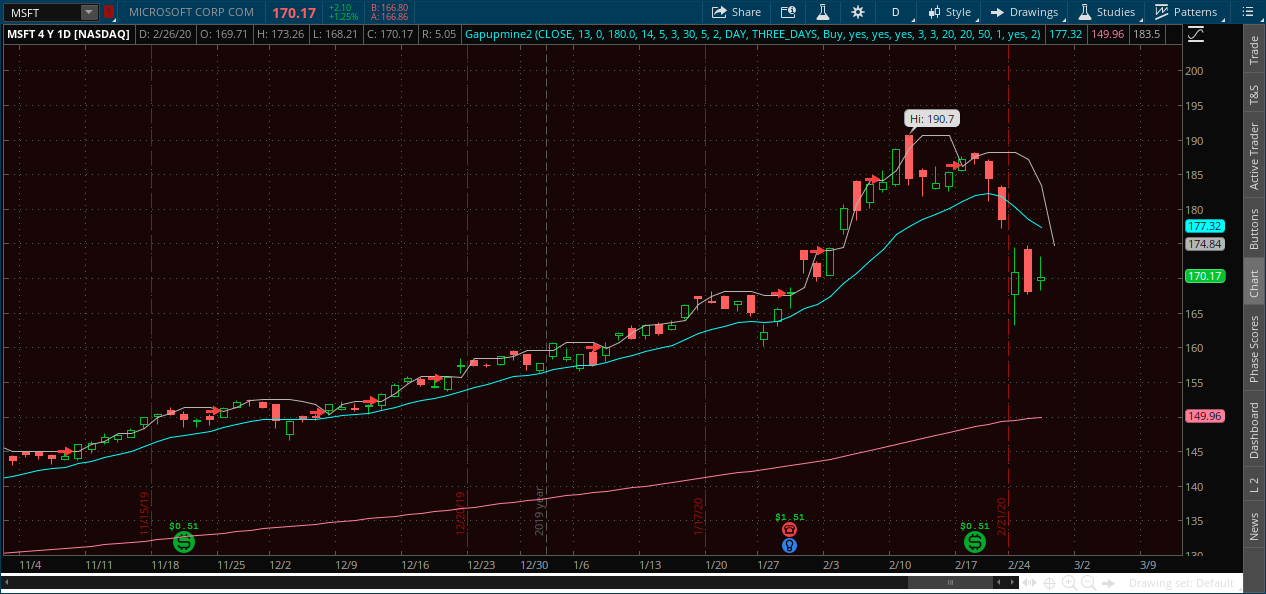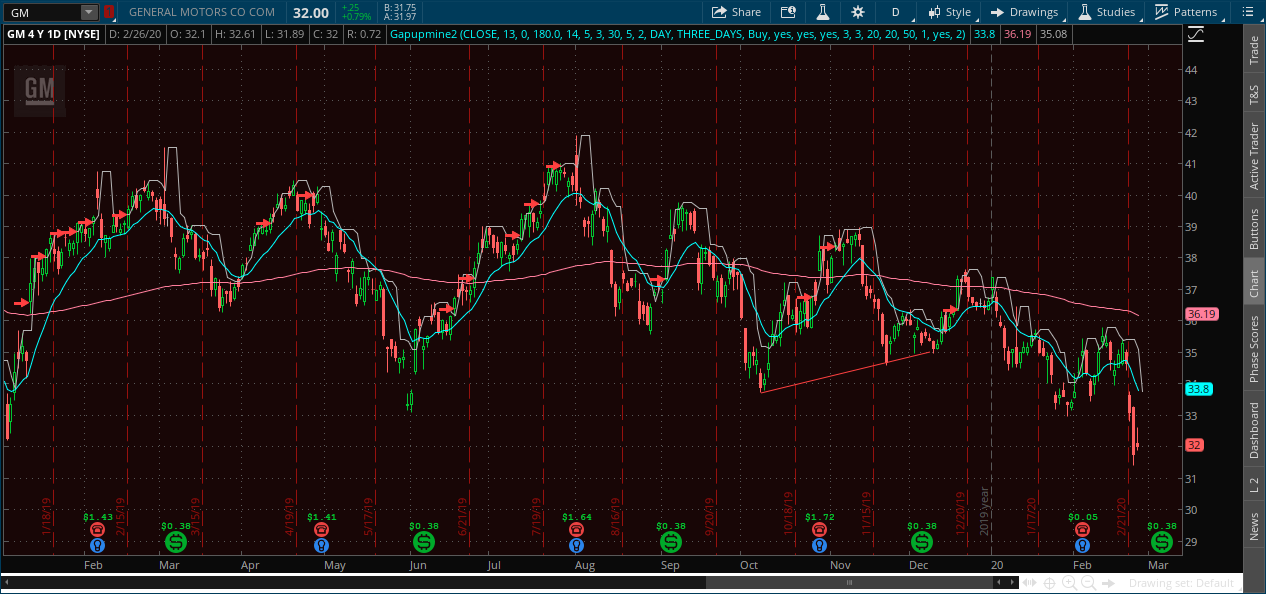# ATR and Exponential Moving Average Divergence Strategy (Ideal for Options)

##### Well-known member
I have developed a strategy that works very well but I can't seem to get the nuances I can pick up with my eyes into the code. Instead I'll share some pictures and explain what to look for. I'd love to hear some ideas for the best way to exit these trades.

Instructions -
1. Only use the strategy on stocks that are in a general longer term uptrend. The best way to determine if it's in an uptrend in my opinion is using your judgement, if you don't trust your judgement pick a longer term ma and only use this strategy when the price is over it.

2. As I'll show in the picture, look for times when the atr goes down or stays flat, but the exp. ma goes up. Make sure the divergence is significant.
Never trade if he atr is below the exp. ma.

3. Enter trades as soon as the high crosses the atr (variable x), this will get you better entries and therefore increase your chance of a better trade.

Disclaimer- Although it wouldn't have an large effect on the results, a divergence atr crosses like the last one on tesla wouldn't be as abvious in the moment because the closing price dragged the close of the exp. ma higher making the divergence seem greater. I tried to account for this somewhat and not include signals that I thought wouldn't be clear in the moment. This effect also happens on a smaller scale on other entries. Personally I still think the Tesla signal would have been detectable because the exp. ma was in a steep uptrend regardless.

Pictures, 2 upward trending stocks, 1 consolidated stock.Code:
``````input price = close;
input length4 = 13;
input displace = 0;
plot AvgExp = ExpAverage(price[-displace], length4);
input length6 = 48.5;
def AvgExp2 = ExpAverage(price[-displace], length6);
def c = close;

input length = 14;
input calcLength = 5;
input smoothLength = 3;
input length2 = 30;
input calclength2 = 5;
input smoothlength2 = 2;
input agg = AggregationPeriod.DAY;
input agg2 = AggregationPeriod.THREE_DAYS;

def zeroline = 0;
def o10 = open(period = agg);
def c10 = close(period = agg);
def data = fold i = 0 to length2
with s
do s + (if c10 > GetValue(o10, i)
then 1
else if c10 < GetValue(o10, i)
then - 1
else 0);
def EMA5 = ExpAverage(data, calclength2);
def Main = ExpAverage(EMA5, smoothLength);
def Signal = ExpAverage(Main, smoothLength);

def zero = if IsNaN(c) then Double.NaN else 0;
def ob = if IsNaN(c) then Double.NaN else Round(length * .7);
def ob2 = if IsNaN(c) then Double.NaN else Round(length * .3);
def  ob3 = if IsNaN(c) then Double.NaN else Round(length * .0504);
def ob4 = if IsNaN(c) then Double.NaN else Round(length * -.7);
def ob5 = if IsNaN(c) then Double.NaN else Round(length * -.3);
def ob6 = if IsNaN(c) then Double.NaN else Round(length * -.2);
def ob7 = if IsNaN(c) then Double.NaN else Round(length * .0);
def os = if IsNaN(c) then Double.NaN else -Round(length * .2);
def os2 = if IsNaN(c) then Double.NaN else -Round(length * .6);
def os3 = if IsNaN(c) then Double.NaN else -Round(length * .0);
def os4 = if IsNaN(c) then Double.NaN else -Round(length * -.1);

def o2 = open(period = agg2);
def c2 = close(period = agg2);
def data2 = fold i2 = 0 to length
with s2
do s2 + (if c2 > GetValue(o2, i2)
then 1
else if c2 < GetValue(o2, i2)
then - 1
else 0);

def EMA52 = ExpAverage(data2, calclength2);
def Main2 = ExpAverage(EMA52, smoothLength);
def Signal2 = ExpAverage(Main2, smoothLength);

input ShowTodayOnly = yes;
input ShowEntryExitBands = yes;
input ShowBubbles = yes;
input SellEntry = 3;
input SellExit = 20;

input ATRLength = 50;
input TargetATRMult = 1;
input DisplayLines = yes;
input PriceDigit = 2;

def today = !ShowTodayOnly or GetDay() == GetLastDay() and SecondsFromTime(0930) >= 0;

# High

def H1  = Highest(high, SellExit);
def H2  = fold i3 = 1 to SellExit
with ip = 0.0
do if GetValue(high, i3) == H1 or GetValue(high, i3) < ip
then ip
else GetValue(high, i3);
def H3  = fold i1 = 1 to SellExit
with ip1 = 0.0
do if GetValue(high, i1) == H1 or GetValue(high, i1) == H2 or GetValue(high, i1) < ip1
then ip1
else GetValue(high, i1);
def HH  = (H2 + H3) / 2.0;

# Low

def L2  = fold i4 = 1 to BuyExit
with ip2 = 10000000.0
do if GetValue(low, i4) == L1 or GetValue(low, i4) > ip2
then ip2
else GetValue(low, i4);
def L3  = Lowest(if low == L1 or low == L2 then 1000000 else low, BuyExit);
def LL  = (L2 + L2) / 2.0;

def QS = Lowest(low, SellEntry);
plot x = QB;
def y = LL;
def ATRVal = ATR(length = ATRLength, averageType = AverageType.SIMPLE);
def mATR = Highest(ATRVal, ATRLength);
def reversethatb = (high - open > 0) and (open > close);

#plot optionbuy = (open > QB) and ((Main - 2 > Main) or (Main > ob)) and (close < QB) and (close < QB) and (close < QB);
#plot optionbuy2 = ((qb <= qb) or (qb < qb)) and (avgexp > avgexp) and (high > qb) and (close > avgexp2);
Ignore everything except the plots x and avgexp.
x is the atr, avgexp in the ma.
Have fun.

•RickAns
with option how would you pick a call or a put with this strategy

Do you have a scan where the 3ema pulls back to the 8ema following price. , thx.

### Not the exact question you're looking for?

87k+ Posts
279 Online## The Market Trading Game Changer

Join 2,500+ subscribers inside the useThinkScript VIP Membership Club
• Exclusive indicators
• Proven strategies & setups
• Private Discord community
• Exclusive members-only content
• 1 full year of unlimited support

What is useThinkScript?

useThinkScript is the #1 community of stock market investors using indicators and other tools to power their trading strategies. Traders of all skill levels use our forums to learn about scripting and indicators, help each other, and discover new ways to gain an edge in the markets.

How do I get started?

We get it. Our forum can be intimidating, if not overwhelming. With thousands of topics, tens of thousands of posts, our community has created an incredibly deep knowledge base for stock traders. No one can ever exhaust every resource provided on our site.

If you are new, or just looking for guidance, here are some helpful links to get you started.

What are the benefits of VIP Membership?